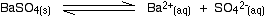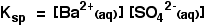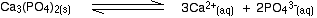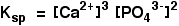AN INTRODUCTION TO SOLUBILITY PRODUCTS

```
```

This page looks at how solubility products are defined, together with their units. It also explores the relationship between the solubility product of an ionic compound and its solubility.

```
```

What are solubility products, Ksp?

Solubility products are equilibrium constants

Barium sulphate is almost insoluble in water. It isn't totally insoluble - very, very small amounts do dissolve. That's true of any so-called "insoluble" ionic compound.

if you shook some solid barium sulphate with water, a tiny proportion of the barium ions and sulphate ions would break away from the surface of the solid and go into solution. Over time, some of these will return from solution to stick onto the solid again.

You get an equilibrium set up when the rate at which some ions are breaking away is exactly matched by the rate at which others are returning.The position of this equilibrium lies very far to the left. The great majority of the barium sulphate is present as solid. In fact, if you shook solid barium sulphate with water you wouldn't be aware just by looking at it that any had dissolved at all.

But it is an equilibrium, and so you can write an equilibrium constant for it which will be constant at a given temperature - like all equilibrium constants.

The equilibrium constant is called the solubility product, and is given the symbol Ksp.To avoid confusing clutter, solubility product expressions are often written without the state symbols. Even if you don't write them, you must be aware that the symbols for the ions that you write are for those in solution in water.```
```

Why doesn't the solid barium sulphate appear in the equilibrium expression?

For many simple equilibria, the equilibrium constant expression has terms for the right-hand side of the equation divided by terms for the left-hand side. But in this case, there is no term for the concentration of the solid barium sulphate. Why not?

This is a heterogeneous equilibrium - one which contains substances in more than one state. In a heterogeneous equilibrium, concentration terms for solids are left out of the expression.

Note:  The simplest explanation for this is that the concentration of a solid can be thought of as a constant. Rather than have an expression with two constants in it (the equilibrium constant and the concentration of the solid), the constants are merged to give a single value - the solubility product.

```
```

Solubility products for more complicated solids

Here is the corresponding equilibrium for calcium phosphate, Ca3(PO4)2:And this is the solubility product expression:Just as with any other equilibrium constant, you raise the concentrations to the power of the number in front of them in the equilibrium equation. There's nothing new here.

```
```

Solubility products only apply to sparingly soluble ionic compounds

You can't use solubility products for normally soluble compounds like sodium chloride, for example. Interactions between the ions in the solution interfere with the simple equilibrium we are talking about.

```
```

The units for solubility products

The units for solubility products differ depending on the solubility product expression, and you need to be able to work them out each time.

Working out the units in the barium sulphate case

Here is the solubility product expression for barium sulphate again:Each concentration has the unit mol dm-3. So the units for the solubility product in this case will be:(mol dm-3) x (mol dm-3)= mol2 dm-6

```
```

Working out the units in the calcium phosphate case

Here is the solubility product expression for calcium phosphate again:The units this time will be:(mol dm-3)3 x (mol dm-3)2= (mol dm-3)5= mol5 dm-15

If you are asked to calculate a solubility product in an exam, there will almost certainly be a mark for the correct units. It isn't very hard - just take care!

```
```

Solubility products apply only to saturated solutions

Let's look again at the barium sulphate case. Here is the equilibrium expression again:. . . and here is the solubility product expression:Ksp for barium sulphate at 298 K is 1.1 x 10-10 mol2 dm-6.

In order for this equilibrium constant (the solubility product) to apply, you have to have solid barium sulphate present in a saturated solution of barium sulphate. That's what the equilibrium equation is telling you.

If you have barium ions and sulphate ions in solution in the presence of some solid barium sulphate at 298 K, and multiply the concentrations of the ions together, your answer will be 1.1 x 10-10 mol2 dm-6.

```
```

What if you mixed incredibly dilute solutions containing barium ions and sulphate ions so that the product of the ionic concentrations was less than the solubility product?

All this means is that you haven't got an equilibrium. The reason for that is that there won't be any solid present. If you lower the concentrations of the ions enough, you won't get a precipitate - just a very, very dilute solution of barium sulphate.

So it is possible to get an answer less than the solubility product when you multiply the ionic concentrations together if the solution isn't saturated.

```
```

Can you get an answer greater than the solubility product if you multiply the ionic concentrations together (allowing for any powers in the solubility product expression, of course)? No!

The solubility product is a value which you get when the solution is saturated. If there is any solid present, you can't dissolve any more solid than there is in a saturated solution.

Note:  In the absence of any solid, a few substances produce unstable supersaturated solutions. As soon as you add any solid, or perhaps just scratch the glass to give a rough bit that crystals can form on, all the excess solid precipitates out to leave a normal saturated solution.

If you mix together two solutions containing barium ions and sulphate ions and the product of the concentrations would exceed the solubility product, you get a precipitate formed. Enough solid is produced to reduce the concentrations of the barium and sulphate ions down to a value which the solubility product allows.

```
```

Summary

• The value of a solubility product relates to a saturated solution.

• If the ionic concentrations give a value less than the solubility product, the solution isn't saturated. No precipitate would be formed.

• If the ionic concentrations give a value more than the solubility product, enough precipitate would be formed to reduce the concentrations to give an answer equal to the solubility product.

```
```
 Questions to test your understanding If this is the first set of questions you have done, please read the introductory page before you start. You will need to use the BACK BUTTON on your browser to come back here afterwards. questions on an introduction to solubility products answers
```
```

Where would you like to go now?

To the solubility product menu . . .

To the Physical Chemistry menu . . .

To Main Menu . . .

```
```

© Jim Clark 2011 (modified November 2013)